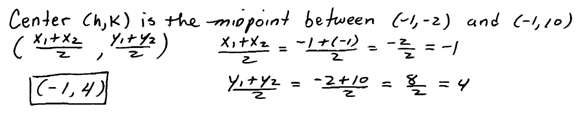Showing posts with label algebra 2. Show all posts
Showing posts with label algebra 2. Show all posts

## Saturday, April 20, 2019

### Free Algebra Study Guide with Videos

This free Algebra study guide is designed to supplement your current textbook. Your textbook is well written in a patient style and the appropriate sections should be read before each class meeting.
 Chapter 1 - Real Numbers and Their Operations    1.1 Real Numbers and The Number Line    1.2 Adding and Subtracting Integers    1.3 Multiplying and Dividing Integers    1.4 Fractions    1.5 Review of Decimals and Percents    1.6 Exponents and Square Roots    1.7 Order of Operations    1.8 Sample Exam Questions Chapter 2 - Linear Equations and Inequalities    2.1 Introduction to Algebra    2.2 Simplifying Algebraic Expressions    2.3 Linear Equations: Part I    2.4 Linear Equations: Part II    2.5 Applications of Linear Equations    2.6 Ratio and Proportion Applications    2.7 Introduction to Inequalities and Interval Notation    2.8 Linear Inequalities (one variable)    2.9 Review Exercises and Sample Exam Chapter 3 - Graphing Lines    3.1 Rectangular Coordinate System    3.2 Graph by Plotting Points    3.3 Graph using Intercepts    3.4 Graph using the y-intercept and Slope    3.5 Finding Linear Equations    3.6 Parallel and Perpendicular Lines    3.7 Introduction to Functions    3.8 Linear Inequalities (Two Variables)    3.9 Review Exercises and Sample Exam Chapter 4 - Solving Linear Systems    4.1 Solving Linear Systems by Graphing    4.2 Solving Linear Systems by Substitution    4.3 Solving Linear Systems by Elimination    4.4 Applications of Linear Systems    4.5 Solving Systems of Linear Inequalities (Two Variables)    4.6 Review Exercises and Sample Exam    [ Elementary Algebra Exam #1 Solutions ]    [ Elementary Algebra Exam #2 Solutions ]    [ Elementary Algebra Exam #3 Solutions ]    [ Elementary Algebra Exam #4 Solutions ]     --- EA Sample Final Exam Ch. 1-7 ---Chapter 5 - Polynomials and Their Operations    5.1 Rules of Exponents (Integer Exponents)    5.2 Introduction to Polynomials and Evaluating    5.3 Adding and Subtracting Polynomials    5.4 Multiplying Polynomials and Special Products    5.5 Dividing Polynomials  (Synthetic Division)    5.6 Negative Exponents and Scientific Notation    5.7 Review Exercises and Sample Exam Chapter 6 - Factoring and Solving by Factoring    6.1 Introduction to Factoring    6.2 Factoring Trinomials    6.3 Factoring Trinomials ax^2 + bx + c    6.4 Factoring Binomials    6.5 General Guidelines for Factoring Polynomials    6.6 Solving Equations by Factoring    6.7 Applications involving Quadratic Equations    6.8 Review Exercises and Sample Exam Chapter 7 - Rational Expressions and Equations    7.1 Simplifying Rational Expressions    7.2 Multiplying and Dividing Rational Expressions    7.3 Adding and Subtracting Rational Expressions    7.4 Complex Fractions    7.5 Solving Rational Equations    7.6 Applications of Rational Equations    7.7 Variation    7.8 Review Exercises and Sample Exam Do you find this cool math site helpful? If so please share the link or embed in your mylab math course. Also, feel free to copy-and-paste anything you find useful here.  All we ask is that you link back to this site:  OpenAlgebra.com

Intermediate Algebra (IA) (Algebra 2) topics below.

## Tuesday, July 23, 2013

### Solving Nonlinear Systems

In this guide, we have solved linear systems using three methods: by graphing, substitution, and elimination.  When solving nonlinear systems, we typically choose the substitution method, however, sometimes the other methods work just as well.  Remember that to solve a system of equations means to find the common points - if they exist. Given a system of equations consisting of a circle and a line then there can be three possibilities for solutions.  Remember that solutions to a system are ordered pairs (x, y). These will be the points where they intersect.
Solve the nonlinear systems.

Given a circle and a parabola there are five possibilities for solutions.
These nonlinear systems given algebraically might look like:
To find the points in common, we will usually use the substitution method. But, as in this case, the graphing and elimination method work just as well.

Solve.

Certainly there are many ways to solve these problems. Experiment with other methods and see if you obtain the same results.

Solve.

---

## Friday, April 26, 2013

### Ellipses

An ellipse is the set of all points whose distances to two fixed points add to the same constant. In this section, we will focus on the equation of ellipses.[InteractiveEllipses ]

The long axis of an ellipse is called the major axis and the short axis is called the minor axis.  These axes terminate at points that we will call vertices.  The vertices along the horizontal axis will be (± a,k) and the vertices along the vertical axes will be (h± b). These points, along with the center, will provide us with a method to sketch an ellipse given standard form.

Given the equation of an ellipse in standard form we can graph it using the following steps:
1. First plot the center (h, k).
2. Plot a point a units up and down from the center.
3. Plot a point b units left and right of the center.
4. Sketch the ellipse and label the four points.In this example the major axis is the vertical axis and the minor axis is the horizontal axis.  The major axis measures 2b = 10 units in length and the minor axis measures 2a = 6 units in length.  There are no x- and y- intercepts in this example.

Graph the ellipse. Label the center and 4 other points.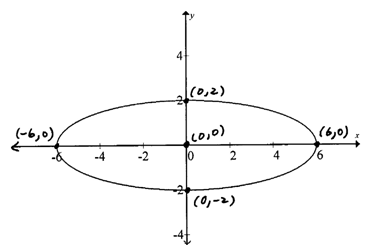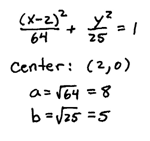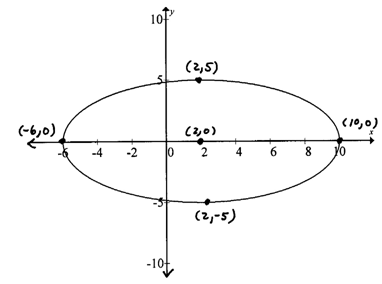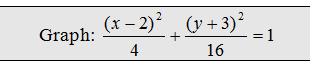It will often be the case that the ellipse is not given in standard form.  In this case, we will begin by rewriting it in standard form.

Graph the ellipse. Label the center and four other points.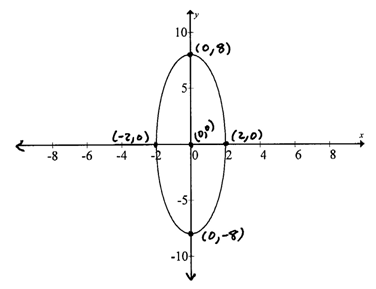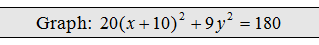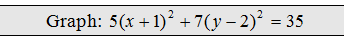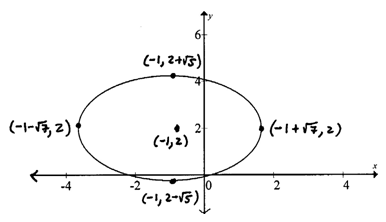The next set of examples give the equations in general form.  Complete the squares to obtain standard form. Remember to factor the leading coefficient out of each variable grouping before using (B/2)^2 to complete the square.

Graph the ellipse. Label the center and four other points.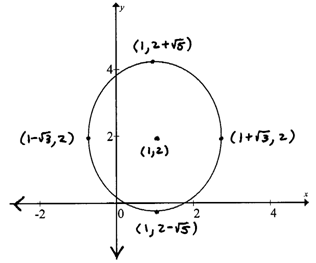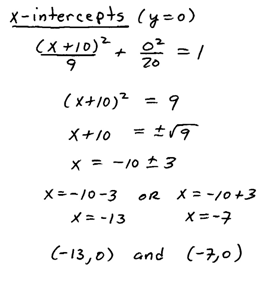When the calculation for intercepts yields complex results then there are no real intercepts.  This just means that the graph does not cross that particular axis.

Example: Find the equation of the ellipse centered at (3, -1) with a horizontal major axis of length 10 units and vertical minor axis of length 4 units.Example: Find the equation of the ellipse given the vertices ( ±3,0) and (0, ±8).Example: Find the equation of the ellipse whose major axis has vertices (-1, -2) and (-1, 10) and minor axis has co-vertices (-3, 4) and (1, 4).